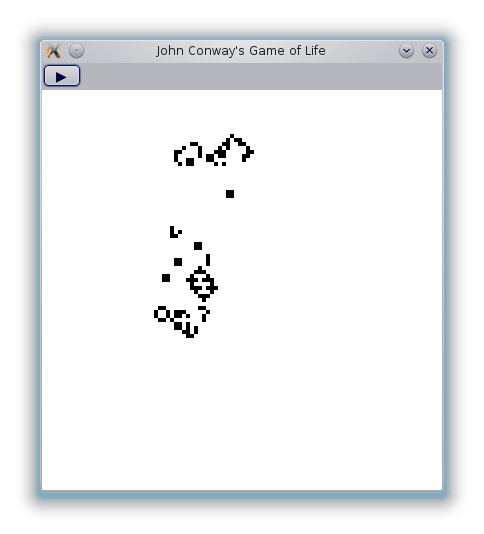## Functional Reactive Programming

Tikhon Jelvis (tikhon@jelv.is)

Zoom in/out: Ctrl or Command + +/-

$$\newcommand{\B}{\mathcal{B}}$$ $$\newcommand{\E}{\mathcal{E}}$$ $$\newcommand{\S}{\mathcal{S}}$$ $$\newcommand{\lb}{\unicode{x27E6}}$$ $$\newcommand{\rb}{\unicode{x27E7}}$$

# 1 Goals

• Program reactive systems declaratively
• Purely functional:
• GUIs
• Controllers
• Music
• Games

# 2 Main Idea

• Make time explicit
• Program with values over time
• Handle both continuous and discrete time

# 3 Behaviors and Events

• Behavior ($$\B$$): value continuous over time (signal)
• Event ($$\E$$): value at a particular time
• Stream ($$\S$$): infinite list of events ordered by time

# 4 Semantics

• Time has to be totally ordered
• Very simple denotational semantics
• $$\lb \B \alpha \rb = \lambda time \to \alpha$$
• $$\lb \E \alpha \rb = time \times \alpha$$
• $$\S\alpha = [\E\alpha ...] = \E(\alpha \times \S\alpha)$$

# 5 Curry-Howard

• Temporal Logic
• Propositions quantified over time
• $$\square x$$ "x always holds" $$\leftrightarrow \B$$
• $$\Diamond x$$ "x will eventually hold" $$\leftrightarrow \E$$
• Modal Logic
• $$\square x \Leftrightarrow \lnot\Diamond\lnot x$$
• $$\Diamond x \Leftrightarrow \lnot\square\lnot x$$

# 6 Dependent Types

• Types based on temporal logic:
• $$\square x \equiv \forall t' \in [t, \infty).\ x_{t'}$$
• $$\B\alpha \equiv \Pi t' : time.\ \alpha_{t'}$$
• $$\Diamond x \equiv \exists t' \in [t, \infty).\ x_{t'}$$
• $$\E\alpha \equiv \Sigma t' : time.\ \alpha_{t'}$$
• Start time consistency

# 7 DemoReactive Banana

# 8 Combinators

• accumB ∷ α → Stream (α → α) → Behavior α
• whenE ∷ Behavior Bool → Stream α → Stream α
• union ∷ Stream α → Stream α → Stream α
• (<$>) ∷ (α → β) → Stream α → Stream β • (<$) ∷ β → Stream α → Stream β

# 9 LifeCode: github.com/TikhonJelvis/Reactive-Life

# 10 Background

• Functions:
• blank ∷ Life
• step ∷ Life → Life
• modify ∷ Point → Life → Life
• Widgets:
• pauseButton
• lifePanel
• timer: event every 100ms

# 11 Input Streams

• time ∷ Stream ()
• from timer
• pauses ∷ Stream ()
• each pauseButton press
• clicks ∷ Stream Point
• each click on lifePanel

# 12 Core Values

active ∷ Behavior Boolean
active = accumB False (not <$pauses) changes ∷ Stream (Life → Life) changes = whenE active (step <$ time) union
modify <$> clicks life ∷ Behavior Life life = accumB blank changes  # 13 Output • sink: bind behavior to a property of a widget sink lifePanel [paint := life] sink pauseButton [text := symb <$> active]
where symb b = if b then "❚❚" else "▶"


# 14 Interface

• Everybody knows and loves monads
• Applicative Functors
• $$\circ x$$ "x will be true at the next time"
• $$x \triangleright y$$ "x will be true then y will be true"